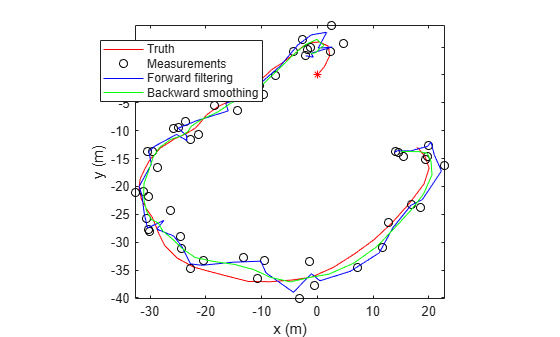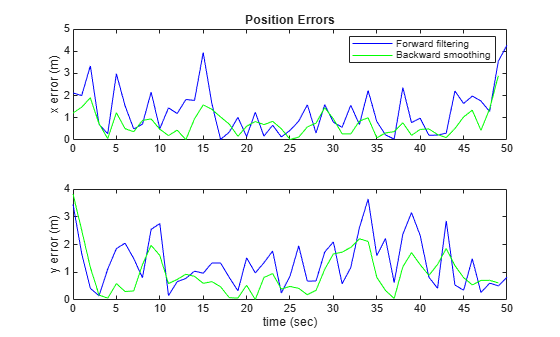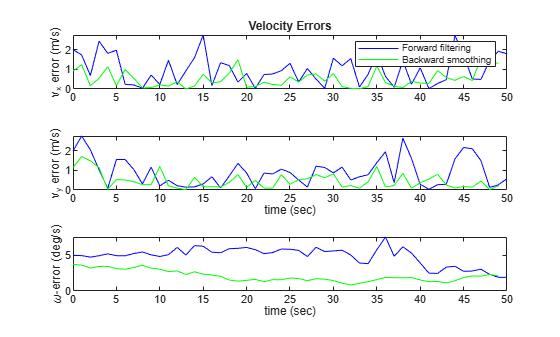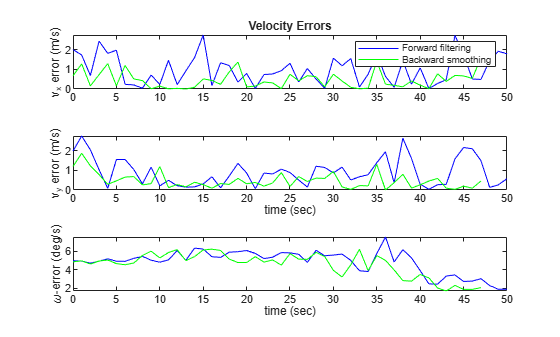# smooth

Backward smooth state estimates of tracking filter

## Syntax

[smoothX,smoothP] = smooth(filter)
[smoothX,smoothP] = smooth(filter,numBackSteps)

## Description

example

[smoothX,smoothP] = smooth(filter) runs a backward recursion to obtain smoothed states and covariances at the previous steps for a tracking filter, filter. The function determines the number of backward steps based on the number of executed forward steps F and the maximum number of backward steps MB specified by the MaxNumSmoothingSteps property of the filter. If F < MB, the number of backward steps is F – 1. Otherwise, the number of backward steps is MB. The number of forward steps is equal to the number of calls to the predict object function of the filter. The backward steps do not include the current time step of the filter.
[smoothX,smoothP] = smooth(filter,numBackSteps) specifies the number of backward smoothing steps numBackSteps. The value of numBackSteps must be less than or equal to the smaller of F – 1 and MB, where F is the number of executed forward steps and MB is the maximum number of backward steps specified by the MaxNumSmoothingSteps property of the filter. 

## Examples

collapse all

Create a truth trajectory based on a constant turn motion model and generate 2-D position measurements.

rng(2020); % For repeatable results % Initialization dt = 1; simTime = 50; tspan = 0:dt:simTime; trueInitialState = [0; 1; 0; 1; 5]; % [x;vx;y;vy;omega] processNoise = diag([0.5; 0.5; 0.1]); % process noise matrix measureNoise = diag([4 4 1]); % measurement noise matrix numSteps = length(tspan); trueStates = NaN(5,numSteps); trueStates(:,1) = trueInitialState; % Propagate the constant turn model and generate the measurements with % noise. for i = 2:length(tspan) trueStates(:,i) = constturn(trueStates(:,i-1),chol(processNoise)*randn(3,1),dt); end measurements = ctmeas(trueStates) + chol(measureNoise)*randn(3,numSteps); 

Plot the truth trajectory and the measurements.

figure plot(trueStates(1,1),trueStates(3,1),'r*','HandleVisibility','off') hold on plot(trueStates(1,:),trueStates(3,:),'r','DisplayName','Truth') plot(measurements(1,:),measurements(2,:),'ko','DisplayName','Measurements') xlabel('x (m)') ylabel('y (m)') axis image

Create a trackingEKF filter object based on the constant turn motion model.

initialGuess = [measurements(1,1);- 1; measurements(2,1); -1; 0]; filter = trackingEKF(@constturn,@ctmeas,initialGuess, ... 'StateCovariance', diag([1,1,1,1,10]), ... 'StateTransitionJacobianFcn',@constturnjac, ... 'MeasurementNoise',measureNoise, ... 'MeasurementJacobianFcn',@ctmeasjac, ... 'EnableSmoothing',true, ... 'MaxNumSmoothingSteps',numSteps); estimateStates = NaN(size(trueStates)); estimateStates(:,1) = filter.State;

Propagate the filter and update the estimated state with the measurements.

for i=2:length(tspan) predict(filter,dt) estimateStates(:,i) = correct(filter,measurements(:,i)); end

Visualize the estimated results.

plot(estimateStates(1,:),estimateStates(3,:),'b','DisplayName','Forward filtering')

Backward smooth the estimated states.

smoothStates = smooth(filter);

Visualize the smoothed trajectory.

plot(smoothStates(1,:),smoothStates(3,:),'g','DisplayName','Backward smoothing') legend('Location','best')Obtain the estimation errors.

forwardError = abs(estimateStates - trueStates); smoothError = abs(smoothStates - trueStates(:,1:end-1)); rmsForward = sqrt(mean(forwardError'.^2))
rmsForward = 1×5 1.6492 1.2326 1.6138 1.1619 5.0195 
rmsSmooth = sqrt(mean(smoothError'.^2))
rmsSmooth = 1×5 0.9201 0.6587 1.2122 0.6139 2.2426 

Visualize the estimation errors. From the results, the smoothing process reduces the estimation errors.

figure subplot(2,1,1) plot(tspan,forwardError(1,:),'b') hold on; plot(tspan(1:end-1),smoothError(1,:),'g') title('Position Errors') legend('Forward filtering','Backward smoothing') ylabel('x error (m)') subplot(2,1,2) plot(tspan,forwardError(3,:),'b') hold on plot(tspan(1:end-1),smoothError(3,:),'g') xlabel('time (sec)') ylabel('y error (m)')figure subplot(3,1,1) plot(tspan,forwardError(2,:),'b') hold on; plot(tspan(1:end-1),smoothError(2,:),'g') title('Velocity Errors') legend('Forward filtering','Backward smoothing') ylabel('v_x error (m/s)') subplot(3,1,2) plot(tspan,forwardError(4,:),'b') hold on; plot(tspan(1:end-1),smoothError(4,:),'g') xlabel('time (sec)') ylabel('v_y error (m/s)') subplot(3,1,3) plot(tspan,forwardError(5,:),'b') hold on; plot(tspan(1:end-1),smoothError(5,:),'g') xlabel('time (sec)') ylabel('\omega-error (deg/s)')Create a truth trajectory based on a constant turn motion model and generate 2-D position measurements.

rng(2020); % For repeatable results % Initialization dt = 1; simTime = 50; tspan = 0:dt:simTime; trueInitialState = [0; 1; 0; 1; 5]; % [x;vx;y;vy;omega] processNoise = diag([0.5; 0.5; 0.1]); % process noise matrix measureNoise = diag([4 4 1]); % measurement noise matrix numSteps = length(tspan); trueStates = NaN(5,numSteps); trueStates(:,1) = trueInitialState; % Propagate the constant turn model and generate the measurements with % noise. for i = 2:length(tspan) trueStates(:,i) = constturn(trueStates(:,i-1),chol(processNoise)*randn(3,1),dt); end measurements = ctmeas(trueStates) + chol(measureNoise)*randn(3,numSteps);

Plot the truth trajectory and the measurements.

figure plot(trueStates(1,1),trueStates(3,1),'r*','HandleVisibility','off') hold on; plot(trueStates(1,:),trueStates(3,:),'r','DisplayName','Truth') plot(measurements(1,:),measurements(2,:),'ko','DisplayName','Measurements') xlabel('x (m)') ylabel('y (m)') axis image;

Create a trackingEKF filter object based on the constant turn motion model. Set the smoothing lag to three steps.

initialGuess = [measurements(1,1);-1;measurements(2,1);-1;0]; filter = trackingEKF(@constturn,@ctmeas,initialGuess,... 'StateCovariance', diag([1,1,1,1,10]),... 'StateTransitionJacobianFcn',@constturnjac,... 'MeasurementNoise', measureNoise,... 'MeasurementJacobianFcn',@ctmeasjac,... 'EnableSmoothing',true,... 'MaxNumSmoothingSteps',4); estimateStates = NaN(size(trueStates)); estimateStates(:,1) = filter.State; stepLag = 3; % Smoothing lag steps smoothStates = NaN(5,numSteps-stepLag);

Propagate the filter and update the estimated state with the measurements.

for i = 2:length(tspan) predict(filter,dt); estimateStates(:,i) = correct(filter,measurements(:,i)); if i > 3 smoothSegment = smooth(filter,stepLag); smoothStates(:,i-3) = smoothSegment(:,1); end end

Visualize the forward estimated and the smoothed trajectories.

plot(estimateStates(1,:),estimateStates(3,:),'b','DisplayName','Forward filtering') plot(smoothStates(1,:),smoothStates(3,:),'g','DisplayName','Backward smoothing') legend('Location','best')Obtain the estimation errors.

forwardError = abs(estimateStates - trueStates); smoothError = abs(smoothStates - trueStates(:,1:end-stepLag)); rmsForward = sqrt(mean(forwardError'.^2))
rmsForward = 1×5 1.6492 1.2326 1.6138 1.1619 5.0195 

Visualize the estimation errors. From the results, the smoothing process reduces the estimation errors.

figure subplot(2,1,1) plot(tspan,forwardError(1,:),'b') hold on plot(tspan(1:end-stepLag),smoothError(1,:),'g') title('Position Errors') legend('Forward filtering','Backward smoothing') ylabel('x error (m)') subplot(2,1,2) plot(tspan,forwardError(3,:),'b') hold on plot(tspan(1:end-stepLag),smoothError(3,:),'g') xlabel('time (sec)') ylabel('y error (m)')figure() subplot(3,1,1) plot(tspan,forwardError(2,:),'b') hold on; plot(tspan(1:end-stepLag),smoothError(2,:),'g') title('Velocity Errors') legend('Forward filtering','Backward smoothing') ylabel('v_x error (m/s)') subplot(3,1,2) plot(tspan,forwardError(4,:),'b') hold on; plot(tspan(1:end-stepLag),smoothError(4,:),'g') xlabel('time (sec)') ylabel('v_y error (m/s)') subplot(3,1,3) plot(tspan,forwardError(5,:),'b') hold on; plot(tspan(1:end-stepLag),smoothError(5,:),'g') xlabel('time (sec)') ylabel('\omega-error (deg/s)')## Input Arguments

collapse all

Filter for object tracking, specified as one of these objects:

Number of backward steps, specified as a positive integer. The value must be less than or equal to the smaller of F – 1 and MB, where F is the number of executed forward steps and MB is the maximum number of backward steps specified by the MaxNumSmoothingSteps property of the filter.

## Output Arguments

collapse all

Smoothed states, returned as an N-by-K matrix. N is the state dimension and K is the number of backward steps. The first column represents the earliest state in the time interval of smoothing, which is the end state of the backward recursion. The last column represents the latest state in the time interval of smoothing, which is the state at the beginning of backward recursion.

Data Types: single | double

Smoothed covariances, returned as an N-by-N-by-K array. N is the state dimension and K is the number of backward steps. Each page (an N-by-N matrix) of the array is the smoothed covariance matrix for the corresponding smoothed state in the smoothX output.

Data Types: single | double

 SÄrkkÄ, Simo. “Unscented Rauch--Tung--Striebel Smoother.” IEEE Transactions on Automatic Control, 53, no. 3 (April 2008): 845–49. https://doi.org/10.1109/TAC.2008.919531.

 Rauch, H. E., F. Tung, and C. T. Striebel. “Maximum Likelihood Estimates of Linear Dynamic Systems.” AIAA Journal 3, no. 8 (August 1965): 1445–50. https://doi.org/10.2514/3.3166.